Author: Oscar Cronquist Article last updated on December 21, 2018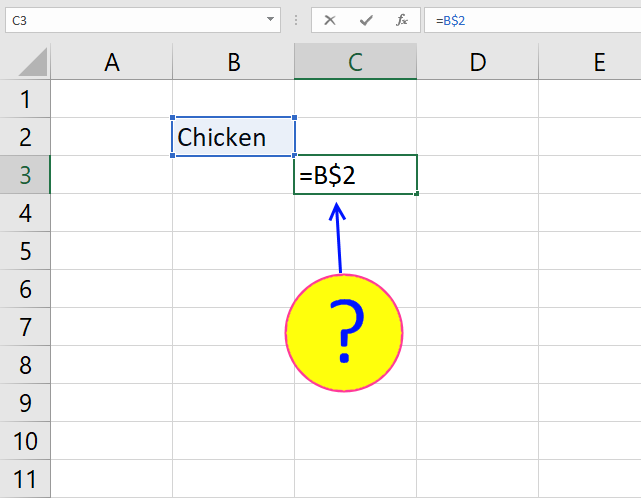What is a reference in Excel? Excel has an A1 reference style meaning columns are named letters A to XFD and rows have numbers, 1 to 1 048 576.  The total number of columns in Excel 2007 are 16384.

A cell can contain a value, formula or constants. A formula starts with a = (equal) sign. You can use values from other cells in your formula. To use another value you create a reference to the cell containing the value you want to use in your formula.

A formula starts with a "=" equal sign. A reference is a column letter and a row number, like B1. B is the column B and 1 is row number one.

Example:

1. Double press with left mouse button on cell A1 and type =B1 then press Enter
2. Double press with left mouse button on cell B1 and type 11 then press Enter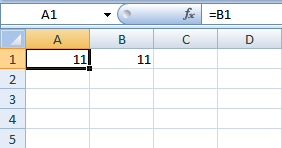You have now created a reference to cell B1. B1 contains the value 11. A1 contains the reference to B1 so both cells now show the number 11. Excel uses relative references by default. =B1 is a relative reference.

### What is a relative reference in excel?

When you copy a cell containing a relative reference and paste it into another cell, the relative reference changes. Let me show you an example.

Now copy cell A1 and paste it to cell A3.

1. Press with right mouse button on on cell A1 and press with left mouse button on Copy
2. Press with right mouse button on on cell A3 and press with left mouse button on Paste
3. Press Escape to remove selection.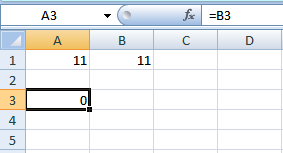When you copy a formula containing relative references the reference changes when you paste it into a another cell. Select cell A3. The formula window now show =B3. See picture above.

Cell A3 has the value 0 (zero) and the formula field shows the relative reference =B3. Cell B3 is empty and that is why cell A3 displays 0 (zero).

When you copied the cell reference in cell A1 to A3, the relative reference changed to B3. The reference changed two rows down. The exact same number as of rows between the copied cell A1 and cell A3.

How much the reference changes is relative to the position of the copied cell and the position of the new cell the formula is pasted into. Excel uses relative references by default.

How to change a relative reference to an absolute reference.1. Double press with left mouse button on A1.
2. Press F4
3. Press Enter

The relative reference =B1 changes to an absolute reference =\$B\$1.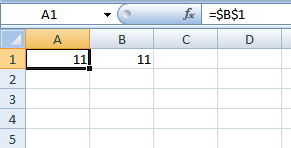### What is an absolute reference in excel?

An absolute reference in a formula stays the same if copied to another cell.

Example:

1. Press with right mouse button on cell A1 and press with left mouse button on Copy
2. Press with right mouse button on cell A3 and press with left mouse button on Paste
3. Press Escape to remove selection.

Select cell A3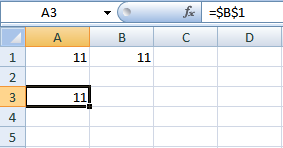The cell reference in cell A3 stays the same (is locked to cell B1). An absolute reference does not change when copied or filled into other cells.

### Insert a row or column

Remember, if you insert a row or column into your excel sheet the absolute reference changes.

Example

Cell A1 contains an absolute reference to B1 (=\$B\$1).

1. Select row 1.
2. Press with right mouse button on on selection and select "Insert".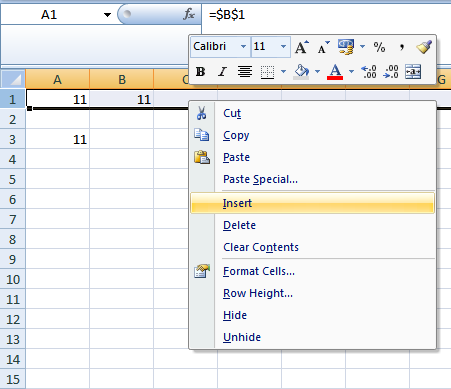3. Done!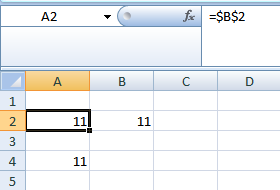The absolute reference changed to =\$B\$2.

To keep absolute references use INDIRECT function.

1. Type =INDIRECT("\$B\$1") + ENTER in cell A1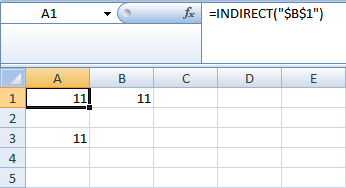2. Select row 1
3. Press with right mouse button on on row 1 and select "Insert"
4. Done!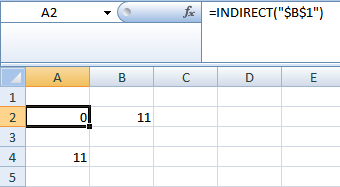The absolute reference is still referencing \$B\$1. \$B\$1 is empty and \$B\$1 returns a 0 (zero).

There is a downside using INDIRECT. INDIRECT is a volatile function. A volatile function is recalculated every time a recalculation is made. You might think that is alright. But if your excel sheet has many calculations and INDIRECT is often used, the calculation can become very slow.